# module4geartrains-190118175406.pdf

23. May 2023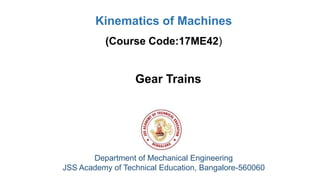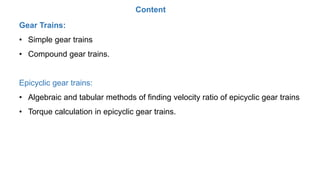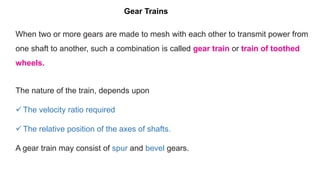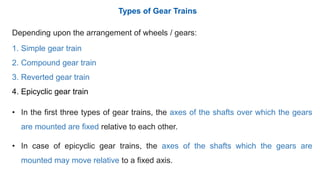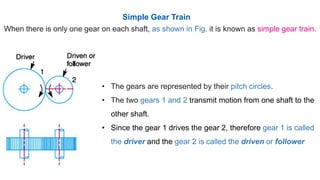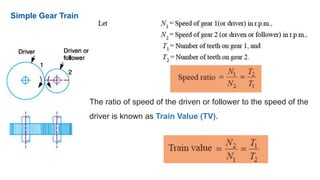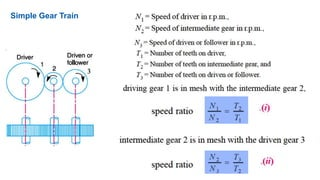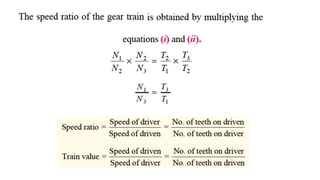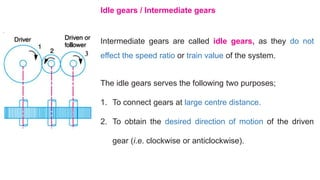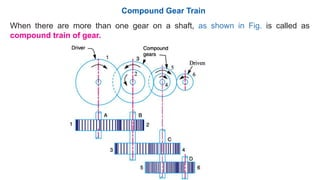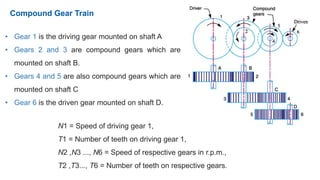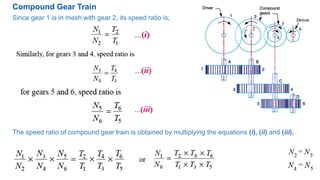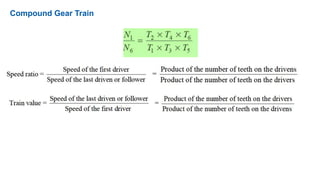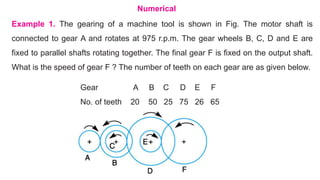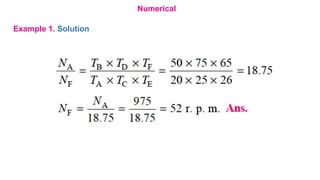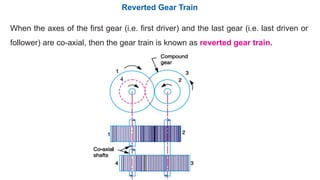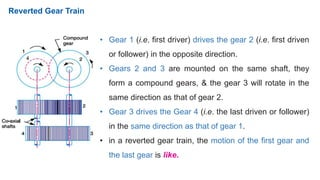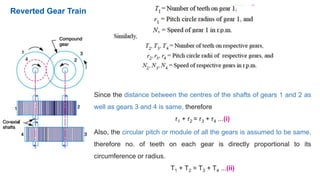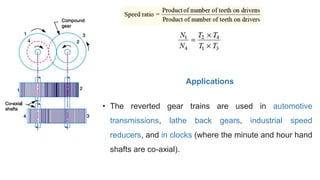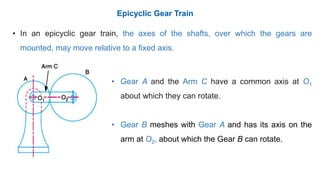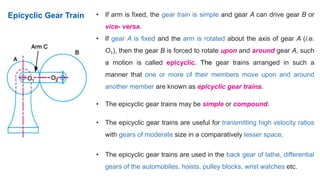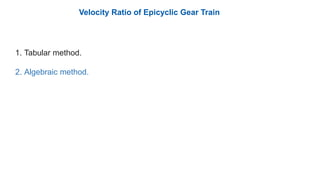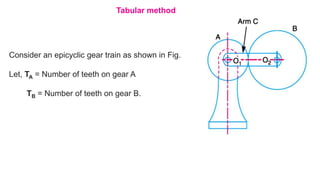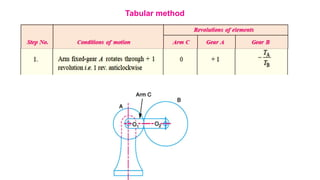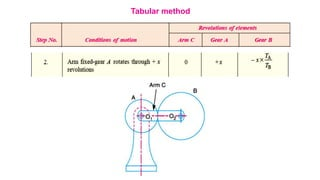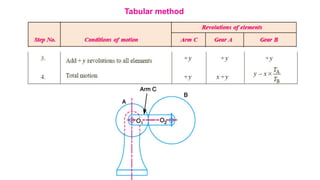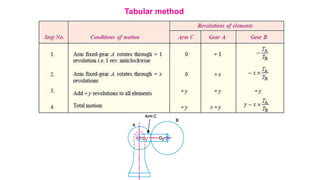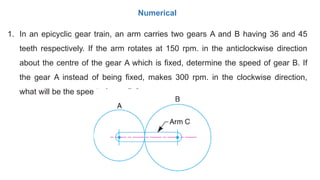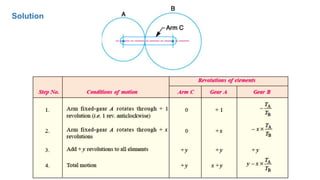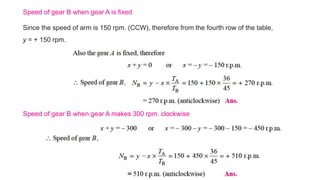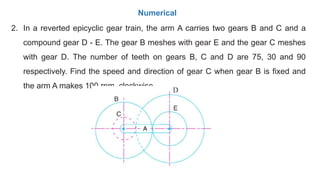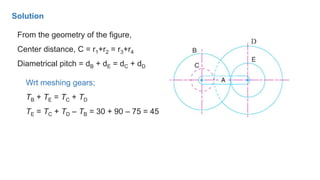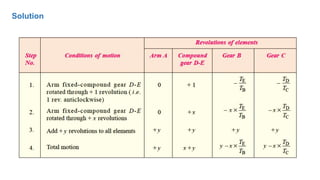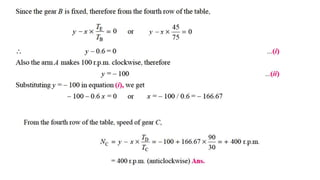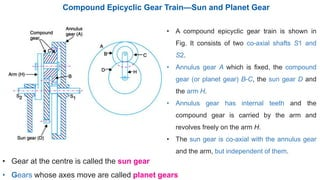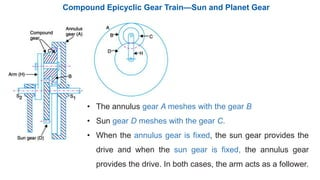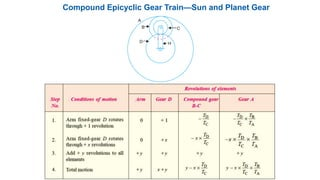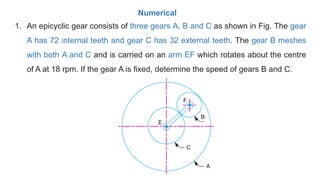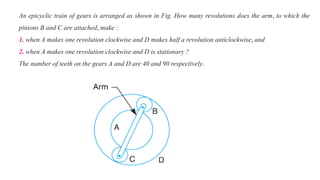1 von 41

### module4geartrains-190118175406.pdf

• 1. Gear Trains Department of Mechanical Engineering JSS Academy of Technical Education, Bangalore-560060 Kinematics of Machines (Course Code:17ME42)
• 2. Gear Trains: • Simple gear trains • Compound gear trains. Epicyclic gear trains: • Algebraic and tabular methods of finding velocity ratio of epicyclic gear trains • Torque calculation in epicyclic gear trains. Content
• 3. When two or more gears are made to mesh with each other to transmit power from one shaft to another, such a combination is called gear train or train of toothed wheels. The nature of the train, depends upon  The velocity ratio required  The relative position of the axes of shafts. A gear train may consist of spur and bevel gears. Gear Trains
• 4. Depending upon the arrangement of wheels / gears: 1. Simple gear train 2. Compound gear train 3. Reverted gear train 4. Epicyclic gear train • In the first three types of gear trains, the axes of the shafts over which the gears are mounted are fixed relative to each other. • In case of epicyclic gear trains, the axes of the shafts which the gears are mounted may move relative to a fixed axis. Types of Gear Trains
• 5. When there is only one gear on each shaft, as shown in Fig. it is known as simple gear train. • The gears are represented by their pitch circles. • The two gears 1 and 2 transmit motion from one shaft to the other shaft. • Since the gear 1 drives the gear 2, therefore gear 1 is called the driver and the gear 2 is called the driven or follower Simple Gear Train
• 6. Simple Gear Train The ratio of speed of the driven or follower to the speed of the driver is known as Train Value (TV).
• 7. Simple Gear Train
• 9. Intermediate gears are called idle gears, as they do not effect the speed ratio or train value of the system. The idle gears serves the following two purposes; 1. To connect gears at large centre distance. 2. To obtain the desired direction of motion of the driven gear (i.e. clockwise or anticlockwise). Idle gears / Intermediate gears
• 10. Compound Gear Train When there are more than one gear on a shaft, as shown in Fig. is called as compound train of gear.
• 11. Compound Gear Train • Gear 1 is the driving gear mounted on shaft A • Gears 2 and 3 are compound gears which are mounted on shaft B. • Gears 4 and 5 are also compound gears which are mounted on shaft C • Gear 6 is the driven gear mounted on shaft D. N1 = Speed of driving gear 1, T1 = Number of teeth on driving gear 1, N2 ,N3 ..., N6 = Speed of respective gears in r.p.m., T2 ,T3..., T6 = Number of teeth on respective gears.
• 12. Since gear 1 is in mesh with gear 2, its speed ratio is; The speed ratio of compound gear train is obtained by multiplying the equations (i), (ii) and (iii), Compound Gear Train
• 13. Compound Gear Train
• 14. Example 1. The gearing of a machine tool is shown in Fig. The motor shaft is connected to gear A and rotates at 975 r.p.m. The gear wheels B, C, D and E are fixed to parallel shafts rotating together. The final gear F is fixed on the output shaft. What is the speed of gear F ? The number of teeth on each gear are as given below. Gear A B C D E F No. of teeth 20 50 25 75 26 65 Numerical
• 15. Numerical Example 1. Solution
• 16. Reverted Gear Train When the axes of the first gear (i.e. first driver) and the last gear (i.e. last driven or follower) are co-axial, then the gear train is known as reverted gear train.
• 17. • Gear 1 (i.e. first driver) drives the gear 2 (i.e. first driven or follower) in the opposite direction. • Gears 2 and 3 are mounted on the same shaft, they form a compound gears, & the gear 3 will rotate in the same direction as that of gear 2. • Gear 3 drives the Gear 4 (i.e. the last driven or follower) in the same direction as that of gear 1. • in a reverted gear train, the motion of the first gear and the last gear is like. Reverted Gear Train
• 18. Reverted Gear Train Since the distance between the centres of the shafts of gears 1 and 2 as well as gears 3 and 4 is same, therefore r1 + r2 = r3 + r4 ...(i) Also, the circular pitch or module of all the gears is assumed to be same, therefore no. of teeth on each gear is directly proportional to its circumference or radius. T1 + T2 = T3 + T4 ...(ii)
• 19. • The reverted gear trains are used in automotive transmissions, lathe back gears, industrial speed reducers, and in clocks (where the minute and hour hand shafts are co-axial). Applications
• 20. Epicyclic Gear Train • In an epicyclic gear train, the axes of the shafts, over which the gears are mounted, may move relative to a fixed axis. • Gear A and the Arm C have a common axis at O1 about which they can rotate. • Gear B meshes with Gear A and has its axis on the arm at O2, about which the Gear B can rotate.
• 21. • If arm is fixed, the gear train is simple and gear A can drive gear B or vice- versa. • If gear A is fixed and the arm is rotated about the axis of gear A (i.e. O1), then the gear B is forced to rotate upon and around gear A, such a motion is called epicyclic. The gear trains arranged in such a manner that one or more of their members move upon and around another member are known as epicyclic gear trains. • The epicyclic gear trains may be simple or compound. • The epicyclic gear trains are useful for transmitting high velocity ratios with gears of moderate size in a comparatively lesser space. • The epicyclic gear trains are used in the back gear of lathe, differential gears of the automobiles, hoists, pulley blocks, wrist watches etc. Epicyclic Gear Train
• 22. Velocity Ratio of Epicyclic Gear Train 1. Tabular method. 2. Algebraic method.
• 23. Tabular method Consider an epicyclic gear train as shown in Fig. Let, TA = Number of teeth on gear A TB = Number of teeth on gear B.
• 24. Tabular method
• 25. Tabular method
• 26. Tabular method
• 27. Tabular method
• 28. 1. In an epicyclic gear train, an arm carries two gears A and B having 36 and 45 teeth respectively. If the arm rotates at 150 rpm. in the anticlockwise direction about the centre of the gear A which is fixed, determine the speed of gear B. If the gear A instead of being fixed, makes 300 rpm. in the clockwise direction, what will be the speed of gear B ? Numerical
• 29. Solution
• 30. Speed of gear B when gear A is fixed Speed of gear B when gear A makes 300 rpm. clockwise Since the speed of arm is 150 rpm. (CCW), therefore from the fourth row of the table, y = + 150 rpm.
• 31. 2. In a reverted epicyclic gear train, the arm A carries two gears B and C and a compound gear D - E. The gear B meshes with gear E and the gear C meshes with gear D. The number of teeth on gears B, C and D are 75, 30 and 90 respectively. Find the speed and direction of gear C when gear B is fixed and the arm A makes 100 rpm. clockwise. Numerical
• 32. From the geometry of the figure, Center distance, C = r1+r2 = r3+r4 Diametrical pitch = dB + dE = dC + dD Wrt meshing gears; TB + TE = TC + TD TE = TC + TD – TB = 30 + 90 – 75 = 45 Solution
• 33. Solution
• 35. Compound Epicyclic Gear Train—Sun and Planet Gear
• 36. Compound Epicyclic Gear Train—Sun and Planet Gear • A compound epicyclic gear train is shown in Fig. It consists of two co-axial shafts S1 and S2. • Annulus gear A which is fixed, the compound gear (or planet gear) B-C, the sun gear D and the arm H. • Annulus gear has internal teeth and the compound gear is carried by the arm and revolves freely on the arm H. • The sun gear is co-axial with the annulus gear and the arm, but independent of them. • Gear at the centre is called the sun gear • Gears whose axes move are called planet gears
• 37. • The annulus gear A meshes with the gear B • Sun gear D meshes with the gear C. • When the annulus gear is fixed, the sun gear provides the drive and when the sun gear is fixed, the annulus gear provides the drive. In both cases, the arm acts as a follower. Compound Epicyclic Gear Train—Sun and Planet Gear
• 38. Compound Epicyclic Gear Train—Sun and Planet Gear
• 39. 1. An epicyclic gear consists of three gears A, B and C as shown in Fig. The gear A has 72 internal teeth and gear C has 32 external teeth. The gear B meshes with both A and C and is carried on an arm EF which rotates about the centre of A at 18 rpm. If the gear A is fixed, determine the speed of gears B and C. Numerical
• 40. An epicyclic train of gears is arranged as shown in Fig. How many revolutions does the arm, to which the pinions B and C are attached, make : 1. when A makes one revolution clockwise and D makes half a revolution anticlockwise, and 2. when A makes one revolution clockwise and D is stationary ? The number of teeth on the gears A and D are 40 and 90 respectively.
• 41. End of Module